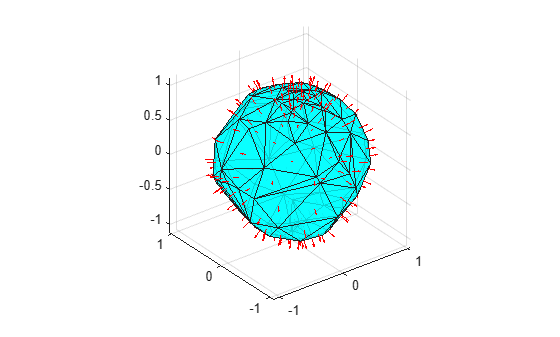# faceNormal

Triangulation unit normal vectors

## Syntax

``F = faceNormal(TR)``
``F = faceNormal(TR,ID)``

## Description

````F = faceNormal(TR)` returns the unit normal vectors to all triangles in a 2-D triangulation. The `faceNormal` function supports 2-D triangulations only. `F` is a three-column matrix where each row contains the unit normal coordinates corresponding to a triangle in `TR.ConnectivityList`.```

example

````F = faceNormal(TR,ID)` returns the unit normal vector to each triangle indexed by `ID`. The identification numbers of the triangles in `TR` are the corresponding row numbers of the property `TR.ConnectivityList`.```

## Examples

collapse all

Compute and plot the unit normal vectors to the facets of a triangulation on a spherical surface.

Create a set of points on a spherical surface.

```rng default; theta = rand([100,1])*2*pi; phi = rand([100,1])*pi; x = cos(theta).*sin(phi); y = sin(theta).*sin(phi); z = cos(phi);```

Triangulate the sphere using the `delaunayTriangulation` function.

`DT = delaunayTriangulation(x,y,z);`

Find the free boundary facets of the triangulation, and use them to create a 2-D triangulation on the surface.

```[T,Xb] = freeBoundary(DT); TR = triangulation(T,Xb);```

Compute the centers and face normals of each triangular facet in `TR`.

```P = incenter(TR); F = faceNormal(TR); ```

Plot the triangulation along with the centers and face normals.

```trisurf(T,Xb(:,1),Xb(:,2),Xb(:,3), ... 'FaceColor','cyan','FaceAlpha',0.8); axis equal hold on quiver3(P(:,1),P(:,2),P(:,3), ... F(:,1),F(:,2),F(:,3),0.5,'color','r');```## Input Arguments

collapse all

Triangulation representation for 2-D triangulations only, specified as a scalar `triangulation` or `delaunayTriangulation` object.

Data Types: `triangulation` | `delaunayTriangulation`

Triangle identification, specified as a scalar or a column vector whose elements each correspond to a single triangle in the triangulation object. The identification number of each triangle is the corresponding row number of the `ConnectivityList` property.

Data Types: `double`